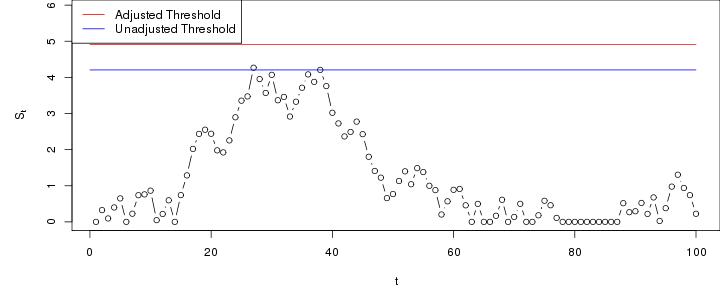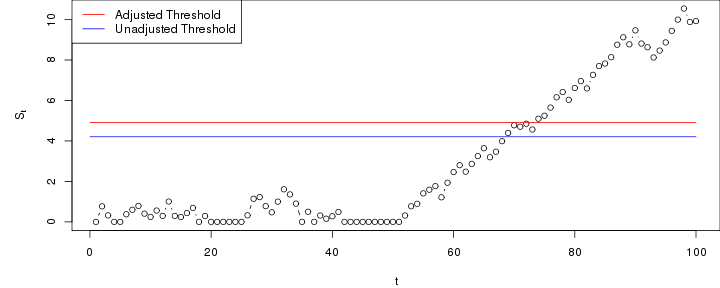# CUSUM Chart based on logistic regression model

In this example we consider an application to CUSUM charts based on a logistic regression models.

Assume we have $$n$$ past in-control data $$(Y_{-n},X_{-n}),\ldots,(Y_{-1},X_{-1})$$, where $$Y_i$$ is a binary response variable and $$X_i$$ is a corresponding vector of covariates.

Suppose that in control $$\mbox{logit}(\mbox{P}(Y_i=1|X_i))=X_i\beta$$. A maximum likelihood estimate $$\hat\beta$$ of the parameters is obtained e.g. by the glm function.

For detecting a change to $$\mbox{logit}(\mbox{P}(Y_i=1|X_i))=\Delta+X_i\beta$$, a CUSUM chart based on the cumulative sum of likelihood ratios of the out-of-control versus in-control model can be defined by (Steiner et al., $$Biostatistics$$ 2000, pp 441-452) $S_0=0, \quad S_t=\max(0, S_{t-1}+R_t)$ where $\exp(R_t)=\frac{\exp(\Delta+X_t\beta)^{Y_t}/(1+\exp(\Delta+X_t\beta))}{\exp(X_t\beta)^{Y_t}/(1+\exp(X_t\beta))} =\exp(Y_t\Delta)\frac{1+\exp(X_t\beta)}{1+\exp(\Delta+X_t\beta)}.$

The following generates a data set of past observations (replace this with your observed past data) from the model $$\mbox{logit}(\mbox{P}(Y_i=1|X_i))=-1+x_1+x_2+x_3$$ and distribution of the covariate values as specified below.

n <- 1000
Xlogreg <- data.frame(x1=rbinom(n,1,0.4), x2=runif(n,0,1), x3=rnorm(n))
xbeta <- -1+Xlogreg$x1+Xlogreg$x2+Xlogreg$x3 Xlogreg$y <- rbinom(n,1,exp(xbeta)/(1+exp(xbeta)))


Next, we initialise the chart and compute the estimate needed for running the chart - in this case $$\hat \beta$$.

library(spcadjust)
chartlogreg <- new("SPCCUSUM",model=SPCModellogregLikRatio(Delta= 1, formula="y~x1+x2+x3"))
xihat <- xiofdata(chartlogreg,Xlogreg)
xihat

##
## Call:  glm(formula = formula, family = binomial("logit"), data = P)
##
## Coefficients:
## (Intercept)           x1           x2           x3
##     -1.0768       1.0341       1.0357       0.9918
##
## Degrees of Freedom: 999 Total (i.e. Null);  996 Residual
## Null Deviance:       1385
## Residual Deviance: 1142  AIC: 1150


## Calibrating the chart to a given average run length (ARL)

We now compute a threshold that with roughly 90% probability results in an average run length of at least 1000 in control. In this regression model this is computed by non-parametric bootstrapping. The number of bootstrap replications (the argument nrep) shoud be increased for real applications.

cal <- SPCproperty(data=Xlogreg,
nrep=100,chart=chartlogreg,
property="calARL",params=list(target=1000),quiet=TRUE)
cal

## 90 % CI: A threshold of 4.906 gives an in-control ARL of at least
##   1000.
## Based on  100 bootstrap repetitions.


## Run the chart

Next, we run the chart with new observations that are in-control.

n <- 100
newXlogreg <- data.frame(x1=rbinom(n,1,0.4), x2=runif(n,0,1), x3=rnorm(n))
newxbeta <- -1+newXlogreg$x1+newXlogreg$x2+newXlogreg$x3 newXlogreg$y <- rbinom(n,1,exp(newxbeta)/(1+exp(newxbeta)))
S <- runchart(chartlogreg, newdata=newXlogreg,xi=xihat)In the next example, the chart is run with data that are out-of-control from time 51 and onwards.

n <- 100
newXlogreg <- data.frame(x1=rbinom(n,1,0.4), x2=runif(n,0,1), x3=rnorm(n))
outind <- c(rep(0,50),rep(1,50))
newxbeta <- -1+newXlogreg$x1+newXlogreg$x2+newXlogreg$x3+outind newXlogreg$y <- rbinom(n,1,exp(newxbeta)/(1+exp(newxbeta)))
S <- runchart(chartlogreg, newdata=newXlogreg,xi=xihat)